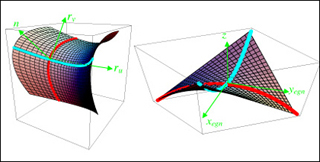3.016 | Fall 2005 | Undergraduate

# Mathematics for Materials Scientists and Engineers

#### Course Description

This course covers the mathematical techniques necessary for understanding of materials science and engineering topics such as energetics, materials structure and symmetry, materials response to applied fields, mechanics and physics of solids and soft materials. The class uses examples from the materials science and …

## Course Info

##### Learning Resource Types
Lecture Notes
Problem Sets with SolutionsParabolic approximation to a surface and local eigenframe. The surface on the left is a second-­order approximation of a surface at the point where the coordinate axes are drawn. The surface has a local normal at that point which is related to the cross product of the two tangents of the coordinate curves that cross at the that point. The three directions define a coordinate system. The coordinate system can be translated so that the origin lies at the point where the surface is expanded and rotated so that the normal n coincides with the z-axis as in the right hand curve. (Image by Prof. W. Craig Carter.)